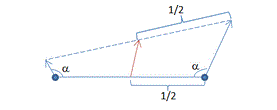Prev: Spaces and Matrices Next: OpenGL skeleton

As discussed in a previous section, the data computed per vertex is interpolated to obtain the fragment data.

The interpolation procedure works perfectly in almost every situation. However, there is a case where interpolation can cause us problems: the interpolation of a normal vector.

Normals in the CG context should be unit length. This is commonly the case when importing a model in our applications, but it is not a requirement. So the normal attribute of a vertex is not guaranteed to be unit length when it arrives at the vertex shader. Furthermore, when transforming a normal vector in the vertex shader, the magnitude of the vector may change. Even if vector n is unit length, there is no guarantee that$n' = normalMatrix \times n$

remains unit length. This can become an issue if interpolating these vectors. Consider the following figure showing interpolated vectors.In the figure, the interpolated vector at the middle should have a vertical direction. As can be seen, the largest vector has more influence on the direction of the interpolated vector.  In the general case, to solve this issue we can normalize the normal vector after transforming it in the vertex shader.

There are however situations where the transformed vector is guaranteed to keep its pre-transformed length. If only translations and rotations are used in the model and view matrices, or to be more precise if these matrices are orthogonal, see the previous section, then the length of the normal vector will be preserved.

In conclusion, we can avoid the normalization of the normal vector in the vertex shader if the normal matrix is orthogonal and we are sure that the application is feeding the vertex shader with normalized vectors. Otherwise, or just to be on the safe side, do normalize the normal vector after transforming it in the vertex shader.

When the vector arrives at the fragment shader we will have to normalize it again! Why? Because interpolation of normalized normal vectors guarantees a good direction, but in the general case the magnitude is wrong! Check the following figure:As can be seen, if the two extreme vectors are unit length, then the middle vector has a smaler magnitude. To solve this we’ll have to normalize the incoming vector in the fragment shader.

Can we avoid this? Yes, but only if the all vertices have the same normal, in which case the interpolated normals will all the equal.

To be on the safe side, always normalize the normal vector in both shaders. In the vertex shader after transforming it, and in the fragment shader before we do anything with it. Beware that the final effect of non-normalizing may not be obvious, so be extra careful when trying to improve performance at the cost of suppressing these normalizations.

All other vectors that can be computed as the difference between two points, see the previous section, do interpolate correctly, hence no normalization is required on the vertex shader.

 Prev: Spaces and Matrices Next: OpenGL skeleton

### 2 Responses to “GLSL Tutorial – Interpolation issues”

1.Did you want to underline the “and”? 🙂

” orthogonal and we are sure that th…”

•Yes 🙁 Fixed. Thanks.

This site uses Akismet to reduce spam. Learn how your comment data is processed.